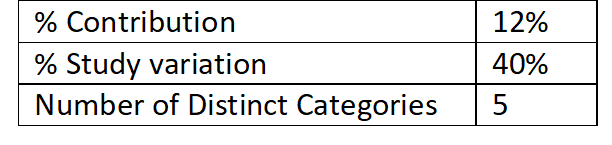India - +91 7838547711, US - +1 513 657 9333

## Lean Six Sigma Black Belt Sample Paper 2: Measure

1. An operational definition:
2. One of the following statements is NOT TRUE:
3. September 23, 1999, after a 286-day journey from Earth to Mars, NASA lost the \$125 million Mars Climate Orbiter, after the spacecraft entered orbit 100 kilometers closer than planned. NASA used the metric system, while its partner Lockheed Martin, the organization that designed the navigation system, used English units. This is a classic example of the absence of____________
4. Measurement value=
5. In case of “Data Collection”, which of the following has the advantage of high data integrity and opportunity to seek clarification?
6. Which of the following is not correctly matched?
7. Formula for DPO is -
8. The correct sequence for conducting Gage R&R study:
9. All the statements below are false, EXCEPT:
10. The following is not an error in Gage R&R study:
11. In case of automated data collection with no human intervention in the measurement,
12. Gage R&R study for a measurement system gave the following outcome, make a suitable inference:13. All the statements below are correct, EXCEPT:
14. One of the following does not warrant an action:
15. While performing Attribute Agreement Analysis (AAA) which of the following is TRUE?
16. The correct sequence for activities given below is:
17. Which of the following combinations is incorrect?
18. A document requires 10 essential entries for further processing. In a sample of 100 such documents, defects (missing entries/incomplete entries/incorrect entries) were evaluated. 20 defects were found in 20 documents. What is DPMO, DPU and Yield respectively?
19. If your data is not normal, then the most appropriate sequence to perform process capability is -

i. Transform the non-normal data to normal data using Box-Cox transformation or Johnsons transformation
ii. Identify the non-normal distribution that fits your data and perform capability analysis for non-normal data
iii. One must investigate the reason for non-normality to check for erroneous data/extreme values
20. Choose the CORRECT one:
21. What is the probability of observing at least one defective in a random sample of size 10, drawn from a population that has been producing, on the average, 10 percent defective units?
22. Errors in invoice’s follow Poisson distribution with DPU of 0.5. What the probability that an invoice will have zero errors?
23. Given process average = 1.65, standard deviation =0.05, assuming statistical control and a normal population, what proportion of the population will meet specifications of 1.65 ± .05?
24. If ZLT=1, what will be ZGOAL if we target 50% reduction in defects?
25. A process that is normally distributed, USL = 24 and LSL = 18. This process has a mean of 20, short-term standard deviation of 1 and long-term standard deviation of 1.5. Calculate Cp, Ppk